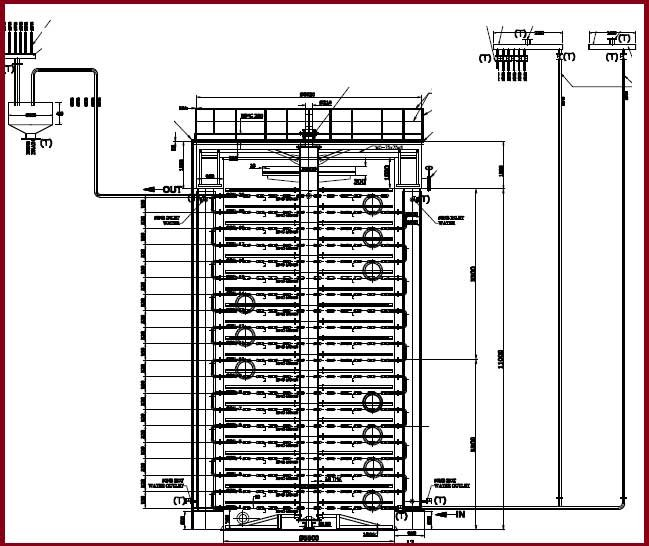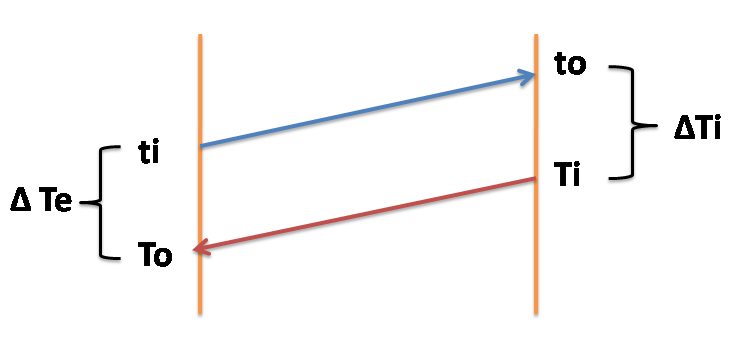# Vertical Crystalliser Design Calculation for Sugar Massecuite Cooling

## Vertical Cooling Crystalliser Design Calculation in Sugar Plant

In the following link covered information  regarding the Vertical crystalliser design

• Vertical crystalliser advantages over the series of crystallisers.
• Types of Vertical cooling crystallisers like Mono Vertical crystalliser (MVC ), Riser type Vertical Crystalliser , Twin Vertical crystalliser.
• Cooling surface requirement for vertical crystalliser ( S/V ratio ).
• Heat Transfer coefficient of Vertical cooling crystalliser.
• Good vertical crystalliser design aspects as per Peter Rein.
• Heat-exchange surface required: (Cooling surface requirement).

• Requirement of heating surface as per formula with example
• Cooling water requirement for crystallizer massecuite cooling
• Mechanical Design of vertical crystalliser – shell thickness and Bottom plate thickness.### Heating Surface Calculation for Vertical Crystalliser

The Basic formula  to find heating surface is

M x Cp x ΔT = S x K x ∆Tm.  Now it can be written as

Heating Surface =S = [M x Cp x ∆T]/ [ K x ∆Tm]

Consider massecuite & cooled water travels in counter-current direction.Here ∆Tm called as logarithmic mean temperature difference

∆T = Ti-To ( Massecuite inlet temperature – Massecuite outlet temperature)∆Ti = Ti-to ( Massecuite inlet temperature  – Water outlet temperature )

∆Te = To-ti ( Massecuite outlet temperature  – Water inlet temperature )

M = Weight of the massecuite in kg/hr = L x V x D

L = Factor taking into account if diluting molasses added for lubrication purpose. Otherwise it will be taken as 1.0

V = Volume of massecuite Lts/hr.

D = specific gravity of the massecuite = 1.5

Cp = specific heat of the massecuite = 0.40 to 0.44 Kcal/kg/oC

K = Heat-transfer coefficient of massecuite.

#### Example:

 ” C ” Massecuite Vertical Crystallizer S. No Description Sign Value UOM Remarks 1 Crushing rate TCH 230 TCH 5000 TCD @ 22 hours basis 2 Massecuite % cane 10 % 3 Weight of the massecuite M 23000 Kg/hr 230 x 10% 4 Specific heat of massecuite Cp 0.44 Kcal/kg/oC 5 Massecuite Inlet temperature Ti 68 oC 6 Massecuite outlet temperature To 40 oC 7 Water inlet temperature ti 32 oC 8 Water outlet temperature to 40 oC 9 Heat transfer coefficient K 25 Kcal/m2/hr/oC 10 S/V ratio 2.0 11 ∆T = Ti – To 28 oC 12 ∆Ti = Ti – to 28 oC 13 ∆Te = To – ti 8 oC 14 Logarithmic mean temperature difference ∆Tm 16.0 oC ∆Ti -∆ Te / ( ln(∆Ti/∆Te)) 15 Heating Surface S 709.97 M2 [M x Cp x ∆T]/ [ K x ∆Tm] 16 Volume of the crystallizers V 355.0 M3

It is better to provide  two no.s of vertical crystallisers with 175 M3 capacity each.

Now it can be calculated in another simple way as follow as

Crushing Rate = 230 TCH

” C ” Massecuite % cane = 8 %

Quantity of massecuite = 18.40 T/hr

Massecuite cooling and ripening time required = 30 hours

Cooling crystallizer capacity required = 18.4 x 30 = 552 MT  = 552 /1.5 = 368 M3

( Here  1.5 = Density of massecuite )

Cooling surface required = 368 / 2 = 184 m2

( Here considered S/V ratio = 2 )

### Cooling water requirement for crystalliser

M x Cp x ΔT =W x Cw x ΔTw

Here

M = Weight of the massecuite in kg/hr

Cp = Specific heat of the massecuite = 0.40 to 0.44 Kcal/kg/oC

∆T = Ti-To ( Massecuite inlet temperature – Massecuite outlet temperature)

W = Weight of the cooled water in kg/hr.

Cw = Specific heat of the water =  1  Kcal/kg/oC

ΔTw = to – ti ( Water outlet temperature – Water inlet temperature)

#### For Example:

 S. No Description Sign Value UOM 1 Weight of the massecuite M 1 Kg/hr 2 Specific heat of massecuite Cp 0.44 Kcal/kg/oC 3 Massecuite Inlet temperature Ti 68 oC 4 Massecuite outlet temperature To 45 oC 5 Water inlet temperature ti 30 oC 6 Water outlet temperature to 40 oC 7 Specific heat of the water Cw 1 Kcal/kg/oC 8 ∆T = Ti-To 23 oC 9 ΔTw = to – ti 10 oC 10 Weight of the cooled water W 1.012 Kg/hr

### Mechanical Design of Vertical cooling crystalliser :

These are mainly calculate shell thickness and Bottom plate thickness

#### Thickness of shell :P = Maximum allowable pressure in kg/cm2

Di = ID of the crystalliser in mm

F = Allowable stress in kg/cm2

J = Welding Joint efficiency in mm

C= corrosion allowance in mm

For example taken

P =  2. 0  kg/cm

Di = 4200 mm ( consider for calculation purpose )

F  = 1400 kg/cm2

J  = 0.75 mm

C = 3 mm

As per above taken values

The thickness of shell = 7 mm

But as per standard specifications  considered 12 mm thickness for crystalliser shell

Some designers  follows another formula as follow as

Some designers considered thickness as per the couress height from the top 16mm/ 12mm/ 10mmHere

µ =  Massecuite density

H = Height of the couress from top in meters

D = Dia of the vertical crystallizer in meters

F = Allowable stress in kg/cm2

J = Welding Joint efficiency in mm

C= corrosion allowance in mm

For example taken (Take total height  Consider as 15 metres  with 10 couress )

µ  =  1.5

H = Height of the couress from top = 15 metres

D = 4.2 metres

F  = 1400 kg/cm2

J  = 0.75 mm

C = 3 mm

According to above formula couress height comes as follow as

Bottom couress thickness = 7.41 mm

Middle couress thickness = 5.16 mm

Top couress thickness = 3.36 mm

But as per standard specifications  considered 16mm / 12 mm / 10mm  thickness for crystallizer shell from bottom to top.

Bottom plate thickness:

Here calculate 250 MT capacity vertical crystalliser bottom plate thickness with 15 meters height and 4 meters Dia

Total momentum of massecuite Mo= M x V / g

M = Total weight of the massecuite = 250 MT = 250 x 106 gm

V = Velocity of inlet massecuite = 0.1 m/sec

g = Gravity factor = 981 cm/sec2

Mo = 250 x 106 x 0.1 / 981 = 25484 gm/cm2

Total load on bottom plate  = weight of the massecuite + momentum of massecuite

= 250 x 106 + 25484

= 250025484 gm

Cross sectional area of the bottom plate  = 0.785 x 400 x 400 = 125600 cm2 ( 4 metres  = 400 cm)

Critical Pressure ( Pc ) = Total load on bottom plate / Cross sectional area of the bottom plate

Critical Pressure ( Pc ) = 250025484 / 125600

= 1990.64 gm/cm2 = 2.0 kg/cm2

Now check the bottom plate thickness by using the following formulaHere

D = Dia of the vertical cooling crystalliser bottom plate in mm = 4000mm

m =  Moment of Inertia = 1 / p

p = Poisson’s ratio  = 0.3

R =  Radius of the bottom plate = 4000 /2 = 2000mm

Es = Modulus factor for MS sheet in kg/cm2    =1.9 x 106 kg/cm2

Pc = Critical Pressure = 2.0 kg/cm2

t = Bottom plate thickness

By substituting all these values in above equation, then get value of ” t “

t = 86.47 mm

But as per standard specification it will be taken 16 mm thickness of bottom plate and it rest on concrete foundation. So concrete foundation will take remaining load.Conversion of units in Sugar Industry Equipment Design Calculations

Sugar Technology – C massecuite purity calculation | C grain dropping and C massecuite final

Role of Bagacillo in vacuum filter | bagacillo cyclone Capacity Calculation

Thumb Rules for Sugar factory Equipment Design Sizing.

Hi friends Thanks for reading. I Hope you liked it.  Give feed back, comments and please share it

## 16 thoughts on “Vertical Crystalliser Design Calculation for Sugar Massecuite Cooling”#### Suryakant Takawane

(June 18, 2018 - 6:45 am)

Useful information for sugar techenologist#### siva alluri

(June 21, 2018 - 2:00 pm)

Thank you Mr.Suryakant Takawane#### javed akhter

(August 25, 2018 - 3:26 am)

very nice and simple that every one can get it#### siva alluri

(August 26, 2018 - 2:52 pm)

Thank you Mr.javed akhter#### Vinayak Mokashi

(August 30, 2018 - 9:46 am)

Thanks, I have no words to express. Fully sugar technology in one roof.#### siva alluri

(September 1, 2018 - 3:38 pm)

Thank you Mr.Vinayak Mokashi#### Aravind

(August 31, 2018 - 1:30 am)

Dear sir
How to calculate the quantity of water used in both B &C and raw sugar Melter example 65 brix#### Anwar

(September 26, 2018 - 10:51 am)

Dear sir
We already have the dates liquid sugar production unit With 1 ton/day.
In that we are looking to have crystallized raw dates sugar approx 500 kg to 1 ton/ day capacity.
In that I hope we need a crystallizer and centrifuge and dryer machine.
We will appreciate you input.
Thank you
Anwar(October 12, 2018 - 9:01 am)

Very nice information#### siva alluri

(October 12, 2018 - 4:07 pm)#### RVV.SATYANARAYANA MURTY

(December 21, 2018 - 8:32 am)

Alluri siva has done a good job what his predecessors neglected.#### siva alluri

(December 23, 2018 - 1:34 pm)

Thank you sir for your valuable appreciation#### Salahuddin Shaikh

(December 22, 2018 - 3:17 am)

Thanks Sir. Your articles are full of knowledge. I do appreciate your efforts for Sugar Mills engineer s guidance. Please send me the calculation of Dearator of a Boiler 1. like the dia of device (tank)

. Dia of spray nozzle 3. Number of spray nozzle. Etc. I shall be very thankful.#### siva alluri

(December 23, 2018 - 1:33 pm)

Ok Mr.Salahuddin Shaikh

We will provide soon